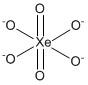QuestionAnswers

# Perxenate ion is:A.$Xe{O_6}^{4 - }$B.$HXe{O_4}^ -$C.$Xe{O_4}^{2 - }$D.$Xe{O_4}^ -$

Hint: Perxenate belongs to compounds of xenon having the valency of xenon more than eight. They are also referred to as hypervalent compounds of xenon. They exhibit octahedral geometry.

Step by step solution:
Perxenates are oxides of xenon compounds. They are the anions having octahedral geometry. They are yellow in colour. The $Xe - O$ bond length is $1.875{\text{ }}{A}$ as measured using X-ray crystallography. The bond angles of $O - Xe - O$ vary in the range of $87^\circ$ to $93^\circ$. The molecular formula of perxenate ion is $Xe{O_6}^{4 - }$ .
The correct answer is A i.e. $Xe{O_6}^{4 - }$. The structure of perxenate ion can be shown as:The valence electrons of $Xe$ are eight so the covalency of $Xe$ is eight. The number of hybrid orbitals is equal to six (six bond pairs + zero lone pairs). Therefore, the hybridization of $Xe{O_6}^{4 - }$ is $s{p^3}{d^2}$. As seen in the structure two oxygen atoms form double and four oxygen atoms form single for fulfilling the octet.
The perxenate ion is produced by treating xenon trioxide with strong alkali.
$2Xe{O_3}(s) + 4O{H^ - }\xrightarrow{{}}Xe(g) + Xe{O_6}^{4 - }(aq) + {O_2}(g) + 2{H_2}O(l)$
Perxenate ions are strong oxidizing agents and can oxidize a variety of metals like silver, copper and manganese. They are reduced to $HXe{O_4}^ -$ in presence of acids.
$HXe{O_4}^ -$ are known as xenates. They are prepared by the reaction of xenon trioxide with aqueous hydroxides. Actually they are the intermediates during the formation of perxenate. The xenon trioxide reacts with hydroxide ion to give xenates which on further reaction with hydroxide ion to yield perxenate.
$Xe{O_3}(aq) + 2O{H^ - }(aq)\xrightarrow{{}}2HXe{O_4}^ - (aq)$
$2HXe{O_4}^ - (aq) + 2O{H^ - }(aq)\xrightarrow{{}}Xe{O_6}^{4 - }(aq) + Xe(aq) + {O_2}(g) + 2{H_2}O(l)$
$Xe{O_4}$ is one of the existing oxides of xenon, but $Xe{O_4}^{2 - }$ and $Xe{O_4}^ -$ do not exist.

Note: Perxenates should not be confused with peroxides of xenon. Perxenate does not contain any peroxide linkages. Perxenates only have single and double bonds with oxygen atoms.